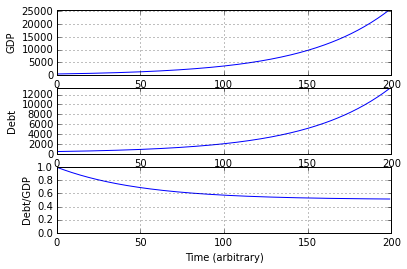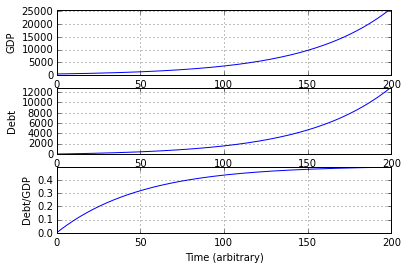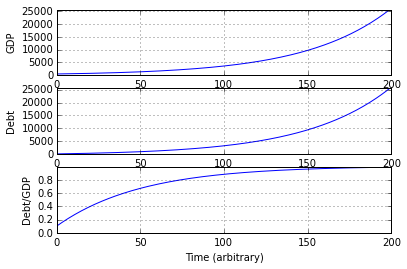# What is a sustainable government deficit?

Some maths and code to understand how growth and deficits affect government debt.

Posted on 14 July 2015 by Andrew Berkeley

A government's budget is in deficit when its expenditure exceeds its tax revenue. Since governments tend to meet this shortfall through a process described as borrowing, deficits add to a government's debt. The UK government is in an almost continual state of budget deficit, with roughly 85% of the post-WWII period being characterised by deficits. This means that UK government debt is continually increasing.

Is this necessarily bad? Is there a sense in which increasing debt is sustainable? Well, if the size of the UK economy is also increasing then the size of the debt relative to the economy may not necessarily be increasing. It is this measure that is usually taken to be more important than the absolute size of the debt.

So here I'll try to figure out how a budget deficit interacts with economic growth to produce an impact on government debt. First I'll develop some simple mathematical descriptions of each of the components (economic growth, debt, etc.) and then produce some simple example scenarios in Python. We'll assume a constant rate of economic growth and a constant rate of deficit spending.

### Economic growth

The size of the economy is usually described by the Gross Domestic Product (GDP) which basically represents the aggregate income of the economy during a particular time period (e.g. a quarter or a year). I'll use the terms GDP and "income" interchangably as labels for the size of the economy. For the sake of simplicity, I'll assume GDP (national income) has a constant growth rate, that is:

$\frac {dI}{dt} = kI$

where $I$ is the national income and $k$ is the growth rate constant. Integrating this gives a simple exponential function:

$I_t = I_0 e^{kt}$

with $I_t$ as the national income at time $t$ and $I_0$ the national income at the start of whatever time period is under consideration. So the economy simply grows at a constant, compounding, rate through time.

It should be noted that, since this is formulated as an exponential function using the base $e$, the growth rate constant is not the same as the more usual type of growth rate expressed as a percentage (e.g. 3%) or decimal fraction (e.g. 0.03). It is, instead, equal to $ln(1+r)$, where $r$ is the growth rate expressed as a decimal fraction. So a growth rate of 3% would be represented by a rate constant of $ln(1 + 0.03) = 0.02955880...$.

### Change in debt

So we have an expression describing how the economy grows through time. Now we need to understand how the debt changes through time. As we stated above, we assume that a government budget deficit adds to the government debt on a pound-for-pound basis, which implies that the government "borrows" when it has a deficit (it could, conceivably, just create money outright). If we describe the deficit relative to GDP as, say, a decimal fraction, and label it $b$, then we can say that the debt ($D$) grows as:

$\frac {dD}{dt} = bI_t$

This simply states that the debt added in any given time period is equal to the deficit over that period (which is a fraction ($b$) of GDP ($I$)). If we integrate this, we get an expression for the variation in debt through time:

$D_t = \frac {b}{k}I_t + C$

where $C$ is the constant of integration. To solve for $C$ we can say that when $t = 0$, $D_t = D_0$ and $I_t = I_0$, and so we can assert that:

$D_0 = \frac {b}{k}I_0 + C$

and therefore:

$C = D_0 - \frac {b}{k}I_o$

The final expression for debt through time is therefore:

$D_t = \frac {b}{k}I_t + D_0 - \frac {b}{k}I_o$

or, alternatively:

$D_t = D_0 + \frac {I_o b}{k} (e^{kt} - 1)$

This expression shows what is intuitively obvious: that the debt at time $t$ is dependent on the current debt ($D_0$) plus the additional debt incurred through time (second term).

### The debt ratio

So we have expressions for how the economy grows and how the government debt changes assuming constant economic growth and deficit rates. Now we can combine them and produce an expression for how the debt ratio - that is the debt relative to GDP - varies with time.

We'll call the debt ratio $D^{R}$, and given the above discussion we can say:

$D^{R}_{t} = \frac {D_t}{I_t}$

Using the first definition for $D_t$ described above we get:

$D^{R}_{t} = \frac {\frac {b}{k}I_t + D_0 - \frac {b}{k}I_o}{I_t}$

which, on separating the denominator among the numerator terms and cancelling, can be rearranged to:

$D^{R}_{t} = \frac {b}{k} + \frac {D_0}{I_t} - \frac {b}{k}\frac{I_o}{I_t}$

and, since $\frac {1}{I_t} = \frac {1}{I_0} e^{-kt}$, simplified to:

$D^{R}_{t} = \frac {b}{k} + (\frac {D_0}{I_0} - \frac {b}{k})e^{-kt}$

So this expression tells us that the debt ratio at any time $t$ depends on the ratio of the deficit rate ($b$) to the growth rate ($k$) and some other term involving an exponential decay function. What we can immediately say about the second term on the right-hand side is that the exponential multiplicand will tend to zero as $t$ tends to infinity. Therefore the entire second term will tend to zero and the debt ratio will converge to the ratio $\frac {b}{k}$. So for any given deficit and growth rates, there is a "target" debt ratio that will be approached that is entirely determined by those respective rates. What the second term in the equation describes is the transition from the starting debt ratio ($\frac {D_0}{I_0}$) to the target ratio.

So, whether or not the debt ratio is increasing or decreasing simply depends on the target debt ratio ($\frac {b}{k}$) and the current debt ratio ($\frac {D_0}{I_0}$). This can be seen by differentiating the expression above:

$\frac {dD^{R}}{dt} = -(\frac {D_0}{I_0} - \frac {b}{k})ke^{-kt}$

This rate of of change will be positive (increasing) or negative (decreasing) depending on the value of the first coefficient - the term inside the parentheses. Specifically, if:

$\frac {D_0}{I_0} > \frac {b}{k}$

then the term in parentheses is positive, the derivative of the debt ratio ($\frac {dD^{R}}{dt}$) will be negative (by virtue of the leading minus sign) and therefore the debt ratio will be decreasing. Alternatively, when:

$\frac {D_0}{I_0} < \frac {b}{k}$

then the term in parentheses is negative, the derivative of the debt ratio will be positive, and the debt ratio will therefore be increasing. In plain language, when the current debt ratio ($\frac {D_0}{I_0}$) is bigger than the target debt ratio implied by the deficit ($b$) and growth rates ($k$) then the debt ratio will be falling, and vice versa.

Clearly the rate of change of the debt ratio will be zero when:

$\frac {b}{k} = \frac {D_0}{I_0}$

which shows that $\frac {b}{k}$ is the equilibrium, or steady-state, ratio.

### Some simulations

Okay, so let's just run a couple of scenarios to visualise the conclusions above.

We'll write a simple bit of Python code to solve the equations above across some time period and plot the results. If we write the solving equations inside a function, we can simply invoke it multiple times with differing arguments without having to duplicate the code.

First thing we need to do is to include some required libraries for handling data vectors and plotting.

In :
import numpy as np
import matplotlib.pyplot as plt
%matplotlib inline


Now we'll write a function which solves the economic growth, debt and debt ratio equations for some specified time period and plots the results.

In :
def run_economy(time_steps, growth_rate, deficit_rate, start_income, start_debt):

# Calculate the rate constant for economy growth based on passed in growth rate
growth_rate_constant = (np.log(1 + growth_rate))

# Initialize a list of timestep indices, e.g. 0, 1, 2, 3,...
# This will be used to solve each of the equations through this number of timesteps
# Since we're using the numpy library we can simply pass this vector of timesteps into
# the equations and get a vector of solutions
time_vector = np.linspace(0,time_steps-1,time_steps)

# Calculate incomes (i.e. GDP with growth) across all timesteps
income = start_income * np.exp(growth_rate_constant * time_vector)

# Calculate debt across all timesteps
debt   = start_debt + (deficit_rate * start_income / growth_rate_constant) * (np.exp(growth_rate_constant * time_vector) - 1)

# Calculate the debt ratio across all timesteps
# We could just divide the debt and income variables at this stage but lets use the elegant form!
debt_ratio = (deficit_rate / growth_rate_constant) + ((start_debt / start_income) - (deficit_rate / growth_rate_constant))*np.exp(-(growth_rate_constant * time_vector))

# Now plot all
fig = plt.figure()

gdp_plot = fig.add_subplot(311, xlim=(0, time_steps), ylim=(0, np.max(income)))
gdp_plot.grid()
gdp_plot.plot(range(time_steps),income)
plt.ylabel('GDP')

debt_plot = fig.add_subplot(312, xlim=(0, time_steps), ylim=(0, np.max(debt)))
debt_plot.grid()
debt_plot.plot(range(time_steps),debt)
plt.ylabel('Debt')

debt_ratio_plot = fig.add_subplot(313, xlim=(0, time_steps), ylim=(0, np.max(debt_ratio)))
debt_ratio_plot.grid()
debt_ratio_plot.plot(range(time_steps),debt_ratio)
plt.ylabel('Debt/GDP')
plt.xlabel('Time (arbitrary)')

fig.show()


Okay, let's invoke this function, passing in some arbitrary arguments

In :
run_economy(200, 0.02, 0.01, 500.0, 500.0)So we solved the equations for 200 timesteps and started with a GDP of £500 and government debt of £500. The debt ratio at the outset was therefore £500/£500 = 1. In other words the value of the government debt was 1 x annual national income. This is indicated in the debt ratio plot in that the line is initially at a value of 1. We specified a growth rate of 2% and a constant deficit spend equal to 1% of GDP. We know from the maths above that the debt ratio should therefore converge to 0.01/0.02 = 0.5, i.e. the value of the government debt should approach 50% of GDP. And as can be seen from the debt ratio plot the line approaches a value of 0.5 as time proceeds. So in this case, a constant deficit of 1% of GDP actually produces a decreasing debt ratio if the growth rate is 2% and the debt ratio at the start is higher than the equlibrium ratio of 0.5.

Let's try another with the same growth and deficit rates, but a different starting point.

In :
run_economy(200, 0.02, 0.01, 500.0, 0.0)So in this case, the same debt ratio is eventually realised, but since the intial debt ratio was zero (we started with no debt), the debt ratio increased to the target value.

Let's try a different target ratio.

In :
run_economy(200, 0.02, 0.02, 500.0, 50.0)In this case, the growth and deficit rates are both equal at 2%. This implies that the equilibrium debt level is 1 x GDP (2%/2% = 1). Since the starting debt ratio is below this (10%) the debt ratio increases through time from 10% to 100%

### Conclusion

A continual deficit is perfectly consistent with a stable or even decreasing debt ratio, depending on the growth rate of the economy. The relative size of the deficit and growth rates determine a target, equilibrium size for the government debt relative to the economy. Whether or not the debt ratio increases or decreases at any given time depends on the current size of the debt ratio relative to the implied target size. When someone complains that the deficit is unsustainable, they need to explain what that means. Do they have a problem with the existing debt level, or the implied target level, or the fact that the debt ratio is increasing or decreasing?

These experiments show that at reasonably realistic levels of economic growth and deficit spending (both in the region of a few percent) the target debt ratio is only realised after many decades. The target ratio is therefore not likley to be realised in practice given that constant rates of economic growth and deficit spending are not likely to persist for decades. But the logic is useful in understanding what impact current or projected growth and deficit rates might have on the debt. At the very least, the equations described here can show whether the debt ratio will increase or decrease at current or forecasted growth and deficit rates. In the case that longer-term, mean growth and deficit rates can be established then the long-term steady-state debt ratio can be identified.

This post was written using the iPython Notebook

The source can be found on Github or viewed in the iPython Notebook Viewer.

The Python code used in this post is also available in script form on Github.